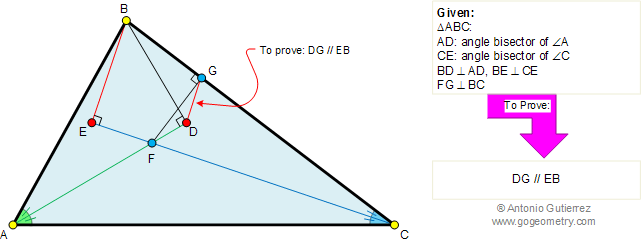Problem 388. Triangle, Angle bisector, Perpendicular, Parallel lines. Level: High School, SAT Prep, College geometry. The figure shows a triangle ABC with angle bisectors AD and CE of angles A and C, respectively. BD is perpendicular to AD and BE is perpendicular to CE. F is the intersection point of AD and CE. If FG is perpendicular to BC, prove that DG is parallel to EB.Recent Additions